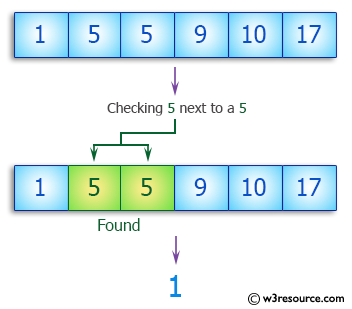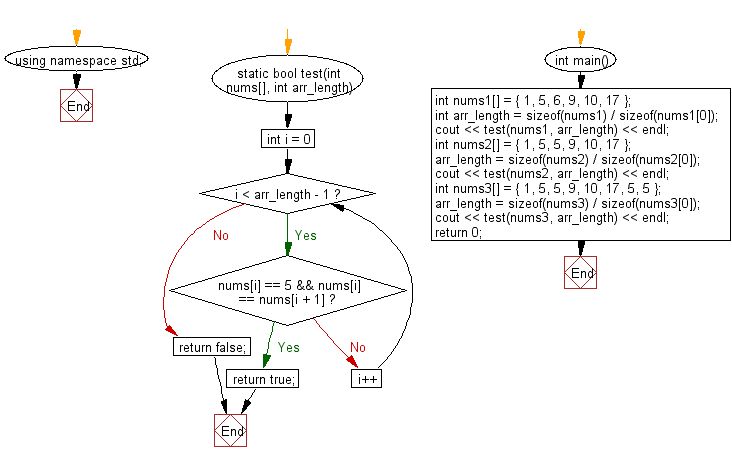﻿ C++ : Check if an array contains 5 next to a 5 somewhere# C++ Exercises: Check if a given array of integers contains 5 next to a 5 somewhere

## C++ Basic Algorithm: Exercise-102 with Solution

Write a C++ program to check if a given array of integers contains 5 next to a 5 somewhere.

Sample Solution:

C++ Code :

``````#include <iostream>
using namespace std;

static bool test(int nums[], int arr_length)
{
for (int i = 0; i < arr_length - 1; i++)
{
if (nums[i] == 5 && nums[i] == nums[i + 1]) return true;
}

return false;
}

int main()
{
int nums1[] = { 1, 5, 6, 9, 10, 17 };
int arr_length = sizeof(nums1) / sizeof(nums1);
cout << test(nums1, arr_length) << endl;
int nums2[] = { 1, 5, 5, 9, 10, 17 };
arr_length = sizeof(nums2) / sizeof(nums2);
cout << test(nums2, arr_length) << endl;
int nums3[] = { 1, 5, 5, 9, 10, 17, 5, 5 };
arr_length = sizeof(nums3) / sizeof(nums3);
cout << test(nums3, arr_length) << endl;
return 0;
}
``````

Sample Output:

```0
1
1
```

Pictorial Presentation:Flowchart:C++ Code Editor: